Electron. J. Differential Equations, Vol. 2020 (2020), No. 85, pp. 1-15.

### Stability of initial-boundary value problem for quasilinear viscoelastic equations Kun-Peng Jin, Jin Liang, Ti-Jun Xiao

Abstract:
We investigate the stability of the initial-boundary value problem for the quasilinear viscoelastic equation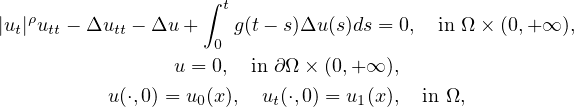where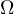is a bounded domain of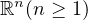with smooth boundary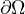,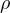is a positive real number, and g(t) is the relaxation function. We present a general polynomial decay result under some weak conditions on g, which generalizes and improves the existing related results. Moreover, under the condition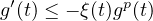, we obtain uniform exponential and polynomial decay rates for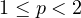, while in the previous literature only the case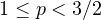was studied. Finally, under a general condition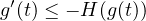, we establish a fine decay estimate, which is stronger than the previous results.

Submitted November 11, 2019. Published July 30, 2020.
Math Subject Classifications: 35Q74, 35B35, 74H55, 74H40, 93D15.
Key Words: Quasilinear viscoelastic equation; polynomial and exponential decay; relaxation function; uniform decay.

Show me the PDF file (357 KB), TEX file for this article.

 Kun-Peng Jin School of Science Chongqing University of Posts and Telecommunications Chongqing 400065, China email: kjin11@fudan.edu.cn Jin Liang School of Mathematical Sciences Shanghai Jiao Tong University Shanghai 200240, China email: jinliang@sjtu.edu.cn Ti-Jun Xiao Shanghai Key Laboratory for Contemporary Applied Mathematics School of Mathematical Sciences Fudan University Shanghai 200433, China email: tjxiao@fudan.edu.cn